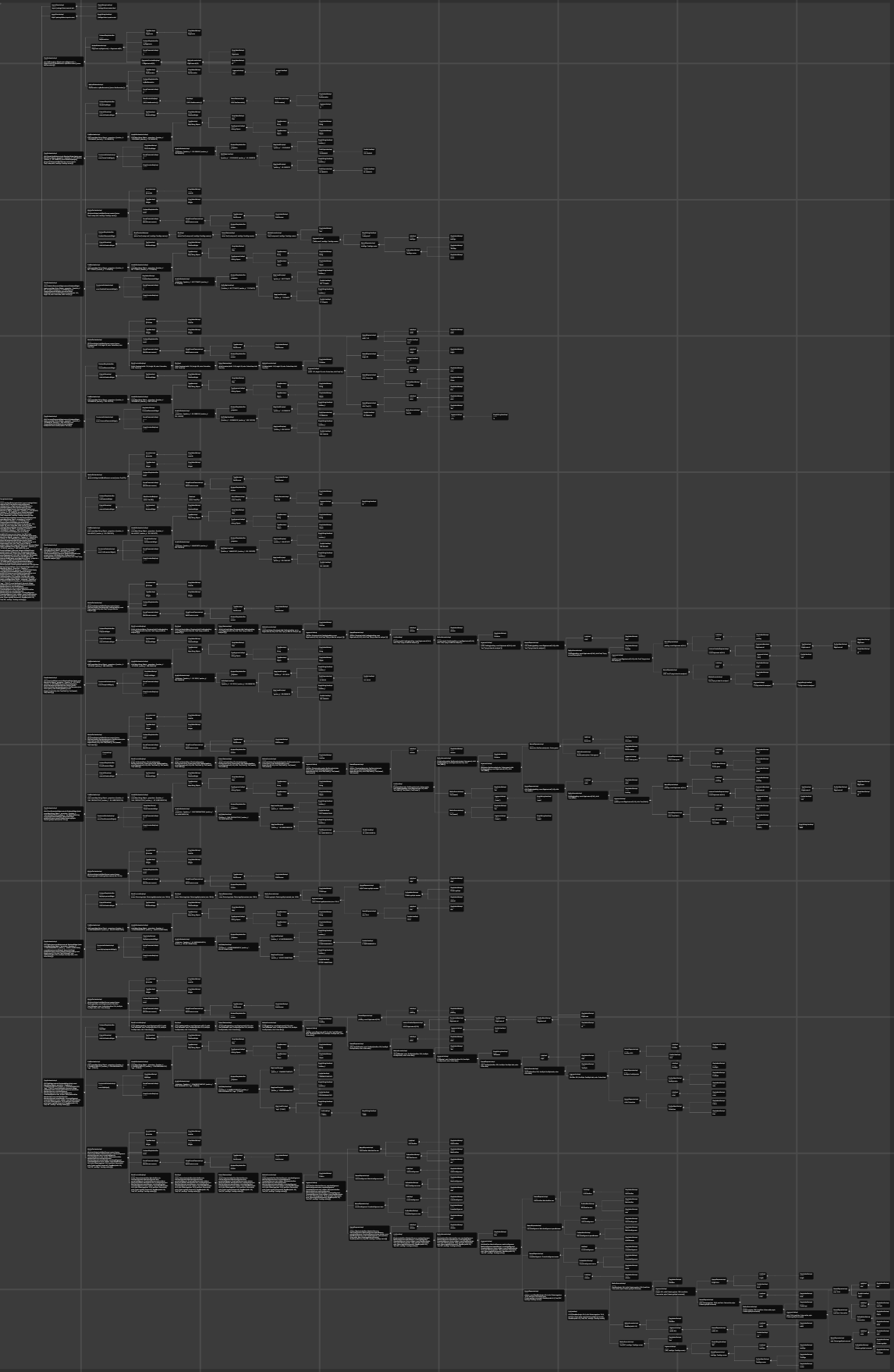# How to use concurrency with data passing without blocking the main isolate.

Please take a look at the following example:

```import 'dart:async';
import 'dart:convert';
import 'dart:math';
import 'package:isolate/isolate.dart';

String str = _generateString();

Future<void> main(List<String> args) async {
final IsolateRunner r = await IsolateRunner.spawn();
final List<Operation> operationAndDuration = [];
const int frameLengthIsMS = 1000 ~/ 120;
final List<int> frames = [];
final Timer timer =
Timer.periodic(const Duration(milliseconds: frameLengthIsMS), (timer) => frames.add(timer.tick));
Stopwatch s;

Future<T> runAsyncOperation<T>(String description, Future<T> Function() operation) async {
s = Stopwatch()..start();
final value = await operation();
return value;
}

T runSyncOperation<T>(String description, T Function() operation) {
s = Stopwatch()..start();
final value = operation();
return value;
}

print("\${str.length} bytes, please wait ~10 seconds.");

final map = await runAsyncOperation("Isolate Decode", () => r.run(decode, str));
final encodedMap = await runAsyncOperation("Isolate Encode", () => r.run(encode, map));

await Future(() {});

final map2 = runSyncOperation("Decode", () => decode(str));
await Future(() {});

final encodedMap2 = runSyncOperation("Encode", () => encode(map2));
await Future(() {});

assert(encodedMap == encodedMap2);

timer.cancel();

print(" • \${_zip(operationAndDuration, findSkippedFrames(frames)).map((v) {
assert(v.value.key == v.key.at);
final dif = v.value.value - v.value.key;
return [
v.key.description,
"Took          : \${v.key.frames}",
"SkippedFrames : \${dif}     (Frame \${v.value.key} to \${v.value.value})",
].join("\n   - ");
}).join("\n\n • ")}");
}

class Operation {
final String description;
final int frames;
final int at;

const Operation(this.description, this.frames, this.at);

@override
String toString() => "Operation '\$description' took '\$frames' frames at '\$at'";
}

List<MapEntry<A, B>> _zip<A, B>(List<A> a, List<B> b) {
assert(a.length == b.length);
return a.asMap().entries.map((entry) => MapEntry(entry.value, b[entry.key])).toList();
}

List<MapEntry<int, int>> findSkippedFrames(List<int> frames) {
final List<MapEntry<int, int>> skippedFrames = [];
frames.fold<int>(null, (previousValue, element) {
if (previousValue == null) return element;
if (previousValue + 1 != element) {
}
return element;
});
return skippedFrames;
}

Map<dynamic, dynamic> decode(String str) => json.decode(str) as Map<dynamic, dynamic>;

String encode(Map<dynamic, dynamic> str) => json.encode(str);

String _generateString() {
final Random rnd = Random();

final Map<dynamic, dynamic> map = <dynamic, dynamic>{};
for (int i = 0; i < 500000; i++) {
map[i.toString()] = [
rnd.nextInt(1000),
(int length) {
final rand = Random();
final codeUnits = List.generate(length, (index) {
return rand.nextInt(33) + 89;
});

return String.fromCharCodes(codeUnits);
}(10)
];
}

return json.encode(map);
}
```

Output:

``````13985982 bytes, please wait ~10 seconds.
• Isolate Decode
- Took          : 196
- SkippedFrames : 152     (Frame 241 to 393)

• Isolate Encode
- Took          : 187
- SkippedFrames : 6     (Frame 482 to 488)

• Decode
- Took          : 47
- SkippedFrames : 47     (Frame 488 to 535)

• Encode
- Took          : 29
- SkippedFrames : 30     (Frame 535 to 565)
``````

I’d like to do expensive operations outside of the main isolate and receive a lot of data (e.g. large decoded json maps) back.

This example is supposed to show that using an isolate to decode a large json would result in 152 skipped frames for this particular run while doing the same work on the main isolate would only cost 47.

How do I decode large JSON strings where

• all of the decoded data is needed in the main isolate (only passing back parts of it is not an option)
• the main isolate is supposed to be able to run smoothly at 120FPS during the whole process.

Related issues: #29480 dart-lang/language#124

## 1 thought on “How to use concurrency with data passing without blocking the main isolate.”

1. Anonymous says: